The `sensobol` package relies on `data.table` and parallel functions in the `boot` and `parallel` package to rapidly compute and bootstrap first, total, and if desired, second and third-order sensitivity indices. It currently uses two estimators: the Jansen (1999) (default) and the Saltelli et al. (2010) estimator.

## Creation of the sample matrix

After loading the package, the first step is to define the settings of the uncertainty and sensitivity analysis, including the sample size of the sample matrix, the number of model inputs and the sensitivity indices we are interested in. As regards to the latter, `sensobol` offers the possibility to calculate up to third-order effects. Once these features are defined, we can build the sample matrix, which relies on Sobol’ quasi-random number sequences, and run the desired model. In this vignette we will use the Sobol’ G function as a test function.

``````library(sensobol)

# Define settings -----------------------------------------

N <- 5000 # Sample size
k <- 8 # Number of parameters
params <- paste("X", 1:8, sep = "") # Vector with the name of the model inputs
R <- 100 # Number of bootstrap replicas

# Create the Sobol' matrices
A <- sobol_matrices(n = N,
k = k,
second = TRUE, # We set a matrix for second-order effects
third = TRUE) # We set a matrix for third-order effects

# Compute model output ------------------------------------
Y <- sobol_Fun(A)``````

## Uncertainty plot

Once the model output is computed, it is informative to visualize its uncertainty. `sensobol` provides a function to assess the model output distribution:

``````
# Plot distribution of model output -----------------------

plot_uncertainty(Y)
#> `stat_bin()` using `bins = 30`. Pick better value with `binwidth`.``````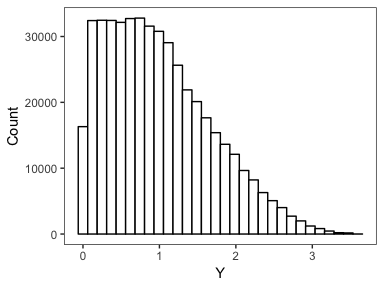## Sensitivity analysis

We can also plot scatterplots of the model output against the model inputs. This is a simple sensitivity analysis that allows to initially observe which model inputs might have an effect on the model output Y, as well as establish its relative importance. In general, scatterplots with “shape” indicate an influential model input.

``````
# Scatterplots of model inputs vs. model output -----------

plot_scatter(x = A,
Y = Y,
n = N,
params = params)``````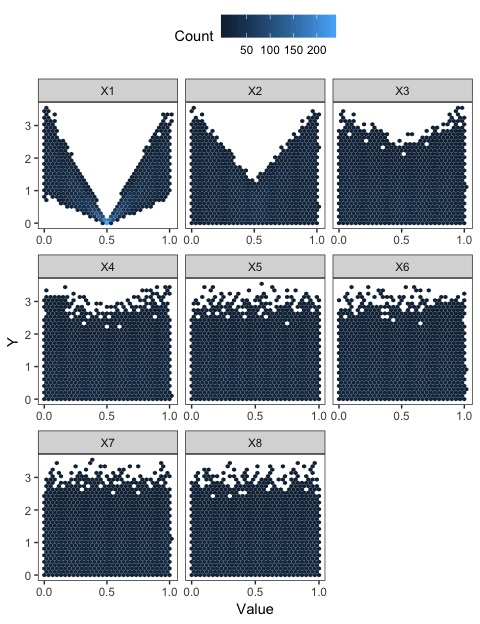We then compute and bootstrap Sobol’ indices. Since we have designed a sample matrix that allows to compute up to third-order effects, we will go for first, second, third and total sensitivity indices here. As stated in the introduction of the vignette, the package currently offers two estimators: the one by Jansen (1999) (default) and the one by Saltelli et al. (2010). The `sobol_indices` function also allows for parallel computing to speed up the bootstrapping. For more information on how to use the `parallel` and `ncpus` parameters, check the `boot` function of the `boot` package.

``````
# Compute Sobol' indices ----------------------------------

dt <- sobol_indices(Y = Y,
params = params,
type = "saltelli",
R = R,
n = N,
second = TRUE,
third = TRUE)``````

The output of `sobol_indices` is a `data.table` with two columns: firstly, the column `parameters`, which indicates whether the Sobol’ indices have been calculated for single model inputs, for pairs of model inputs (second-order) or for three model inputs (third-order). Secondly, the column `V1`, which stores an object of class `boot` with all the results of the bootstrap. Column `V1`, for instance, stores the bootstrap replicas. This is useful if we want to assess their distribution in order to select the most appropriate method for the computation of confidence intervals:

``````
# Show output of the sobol_indices function ---------------

print(dt)
#>     parameters     V1
#>  1:         X1 <boot>
#>  2:         X2 <boot>
#>  3:         X3 <boot>
#>  4:         X4 <boot>
#>  5:         X5 <boot>
#>  6:         X6 <boot>
#>  7:         X7 <boot>
#>  8:         X8 <boot>
#>  9:      X1.X2 <boot>
#> 10:      X1.X3 <boot>
#> 11:      X1.X4 <boot>
#> 12:      X1.X5 <boot>
#> 13:      X1.X6 <boot>
#> 14:      X1.X7 <boot>
#> 15:      X1.X8 <boot>
#> 16:      X2.X3 <boot>
#> 17:      X2.X4 <boot>
#> 18:      X2.X5 <boot>
#> 19:      X2.X6 <boot>
#> 20:      X2.X7 <boot>
#> 21:      X2.X8 <boot>
#> 22:      X3.X4 <boot>
#> 23:      X3.X5 <boot>
#> 24:      X3.X6 <boot>
#> 25:      X3.X7 <boot>
#> 26:      X3.X8 <boot>
#> 27:      X4.X5 <boot>
#> 28:      X4.X6 <boot>
#> 29:      X4.X7 <boot>
#> 30:      X4.X8 <boot>
#> 31:      X5.X6 <boot>
#> 32:      X5.X7 <boot>
#> 33:      X5.X8 <boot>
#> 34:      X6.X7 <boot>
#> 35:      X6.X8 <boot>
#> 36:      X7.X8 <boot>
#> 37:   X1.X2.X3 <boot>
#> 38:   X1.X2.X4 <boot>
#> 39:   X1.X2.X5 <boot>
#> 40:   X1.X2.X6 <boot>
#> 41:   X1.X2.X7 <boot>
#> 42:   X1.X2.X8 <boot>
#> 43:   X1.X3.X4 <boot>
#> 44:   X1.X3.X5 <boot>
#> 45:   X1.X3.X6 <boot>
#> 46:   X1.X3.X7 <boot>
#> 47:   X1.X3.X8 <boot>
#> 48:   X1.X4.X5 <boot>
#> 49:   X1.X4.X6 <boot>
#> 50:   X1.X4.X7 <boot>
#> 51:   X1.X4.X8 <boot>
#> 52:   X1.X5.X6 <boot>
#> 53:   X1.X5.X7 <boot>
#> 54:   X1.X5.X8 <boot>
#> 55:   X1.X6.X7 <boot>
#> 56:   X1.X6.X8 <boot>
#> 57:   X1.X7.X8 <boot>
#> 58:   X2.X3.X4 <boot>
#> 59:   X2.X3.X5 <boot>
#> 60:   X2.X3.X6 <boot>
#> 61:   X2.X3.X7 <boot>
#> 62:   X2.X3.X8 <boot>
#> 63:   X2.X4.X5 <boot>
#> 64:   X2.X4.X6 <boot>
#> 65:   X2.X4.X7 <boot>
#> 66:   X2.X4.X8 <boot>
#> 67:   X2.X5.X6 <boot>
#> 68:   X2.X5.X7 <boot>
#> 69:   X2.X5.X8 <boot>
#> 70:   X2.X6.X7 <boot>
#> 71:   X2.X6.X8 <boot>
#> 72:   X2.X7.X8 <boot>
#> 73:   X3.X4.X5 <boot>
#> 74:   X3.X4.X6 <boot>
#> 75:   X3.X4.X7 <boot>
#> 76:   X3.X4.X8 <boot>
#> 77:   X3.X5.X6 <boot>
#> 78:   X3.X5.X7 <boot>
#> 79:   X3.X5.X8 <boot>
#> 80:   X3.X6.X7 <boot>
#> 81:   X3.X6.X8 <boot>
#> 82:   X3.X7.X8 <boot>
#> 83:   X4.X5.X6 <boot>
#> 84:   X4.X5.X7 <boot>
#> 85:   X4.X5.X8 <boot>
#> 86:   X4.X6.X7 <boot>
#> 87:   X4.X6.X8 <boot>
#> 88:   X4.X7.X8 <boot>
#> 89:   X5.X6.X7 <boot>
#> 90:   X5.X6.X8 <boot>
#> 91:   X5.X7.X8 <boot>
#> 92:   X6.X7.X8 <boot>
#>     parameters     V1``````

We can access and check the bootstrap replicas of the first and total-order indices as follows:

``````
# Access boot replicas ------------------------------------

# Copy data table
b.rep <- data.table::copy(dt)

# Retrieve
tmp1 <- b.rep[1:k, lapply(V1, function(x) x[["t"]])][
, sensitivity:= rep(c("Si", "STi"), each = R)
]

# Melt
temp2 <- data.table::melt(tmp1, measure.vars = 1:k)

# Plot
plot_uncertainty(temp2[, value]) +
ggplot2::facet_wrap(temp2[, sensitivity]~temp2[, variable],
scales = "free_x") +
ggplot2::labs(x = "Variance",
y = "Count") +
ggplot2::scale_x_continuous(breaks = scales::pretty_breaks(n = 3))
#> `stat_bin()` using `bins = 30`. Pick better value with `binwidth`.``````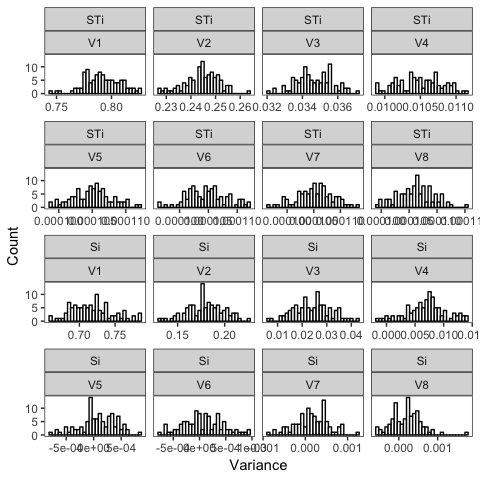Distribution of the bootstrap replicas.

Once we have assessed the distribution of the replicas, we can decide which confidence interval suits our data best. `sensobol` currently offers suport for normal, percentile, bca and basic confidence intervals, all retrieved internally from the `boot::boot.ci` function. In this case, we will compute normal confidence intervals:

``````
# Compute confidence intervals ----------------------------

dt.ci <- sobol_ci(dt,
params = params,
type = "norm",
conf = 0.95,
second = TRUE,
third = TRUE) ``````

### Calculation of the approximation error

Due to measurement error, it is likely that model inputs that are not influential display sensitivity indices that are not zero. In order to assess the extent of this measurement error, we can calculate the Sobol’ indices of a dummy parameter. This allows to differentiate truly influential model inputs from those whose non-zero indices might simply result from the noise of the calculation. The `sensobol` package implements the approach by Khorashadi Zadeh et al. (2017) to calculate and bootstrap the Sobol’ indices of a dummy parameter:

``````
# Compute Sobol' indices of a dummy parameter -------------

dt.dummy <- sobol_dummy(Y = Y,
params = params,
R = R,
n = N)``````

The function `sobol_dummy_ci` computes the confidence intervals for the Sobol’ indices of the dummy parameter:

``````
# Compute confidence intervals for the dummy parameter ----

dt.dummy.ci <- sobol_ci_dummy(dt.dummy,
type = "norm",
conf = 0.95)``````

To visualize Sobol’ first (\(S_i\)) and total (\(S_{Ti}\)) -order indices, use the `plot_sobol` function with `type = 1`. This setting also allows to plot the confidence intervals of the dummy parameter, if needed. For second (\(S_{ij}\)) and third (\(S_{ijk}\)) - order indices, use `type = 2` and `type = 3` respectively. Narrower confidence intervals will be obtained by designing a sample matrix with a larger sample size N.

``````
# Plot Sobol' first and total-order indices -------------------------

plot_sobol(dt.ci, dummy = dt.dummy.ci, type = 1)``````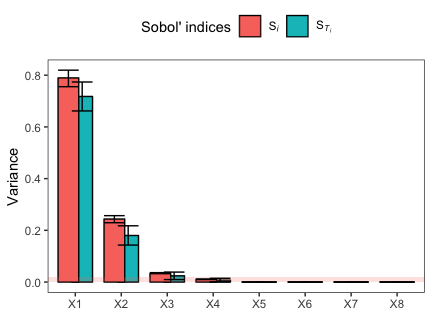First and total-order Sobol’ indices. The transparent, blue horizontal frame shows the approximation error for \(S_{Ti}\), computed via a dummy parameter. Only the model inputs whose lower confidence interval for \(S_{Ti}\) do not overlap the frame can be considered to truly have an interaction effect. The approximation error for \(S_i\) is very small and can not be seen in the plot.

``````
# Plot Sobol' second and third-order indices ------------------------

lapply(2:3, function(x) plot_sobol(dt.ci, type = x))
#> []``````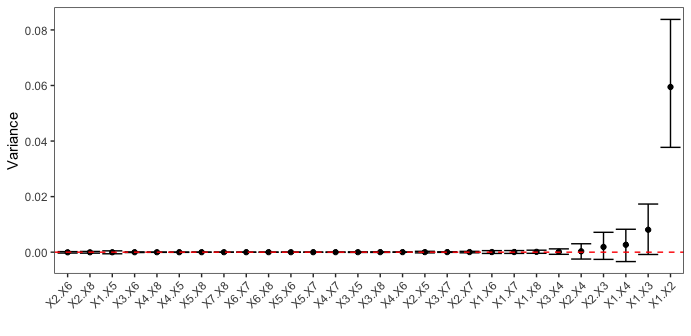``````#>
#> []``````